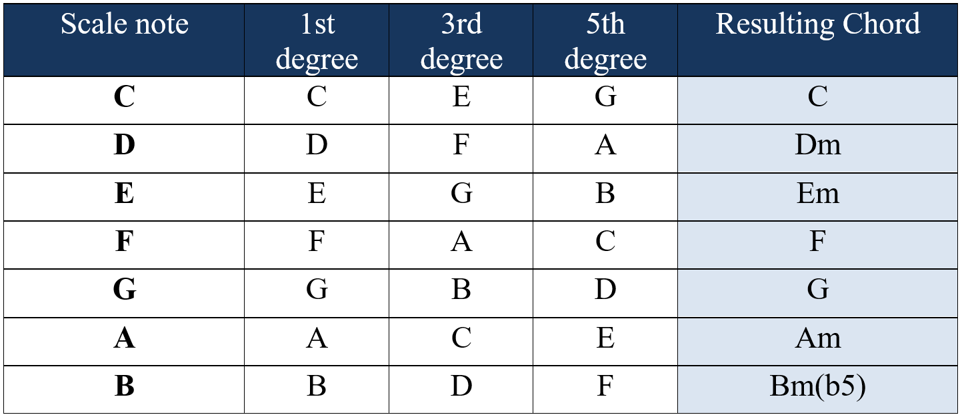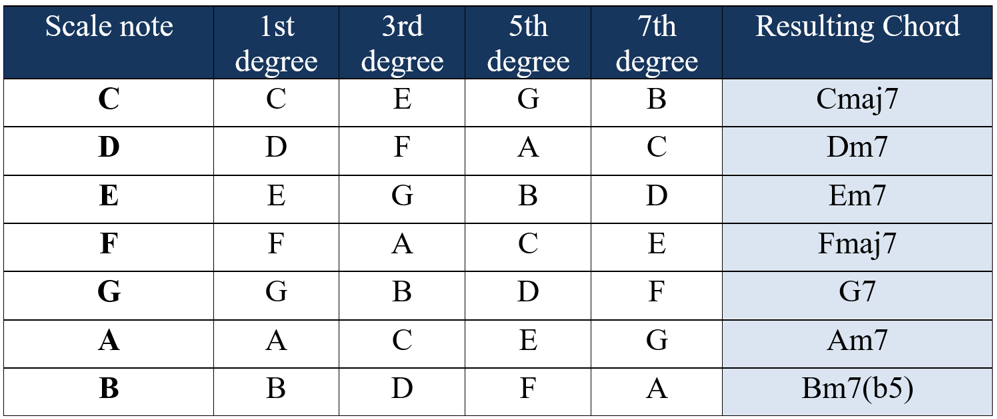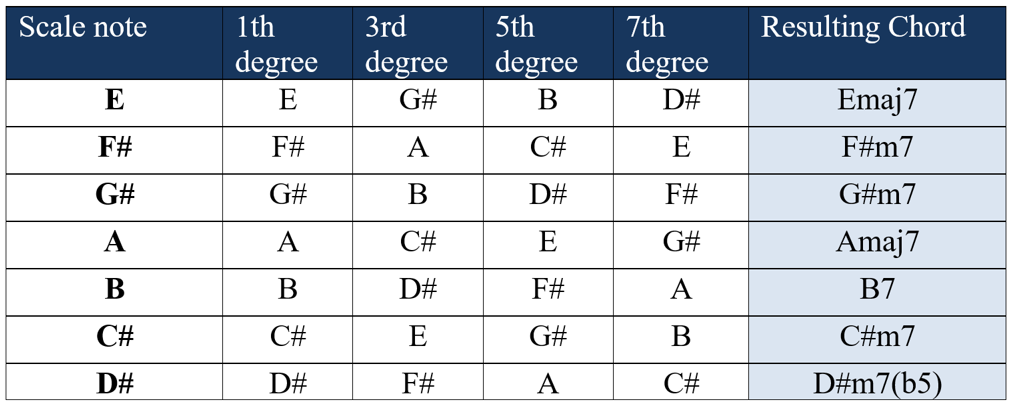# Tonality – Chords of a key

Chords of a key are chords formed from a given scale. Take the C major scale as an example: C, D, E, F, G, A, B.

For each note of this scale, we will form a chord. Therefore, we will have seven chords, which will be the chords of the key of C major. How do we do this?

For each note of the scale, the respective chord will be formed using the first, the third and the fifth degrees (counted from that note, on top of that same scale). Let’s start with the note C. The first degree is C. The third degree, counting from C, is E. The fifth degree, counting from C, is G.

The first chord of the key of C major is then formed by the notes C, E, G (notice that this is the C major chord, since E is the major third of C).

## Building the second chord of a major key

Now let’s build the chord of the next note on the scale, which is D. The first degree is D. The third degree, counting from D, on this scale, is F. The fifth degree, counting from D, is A. Therefore, the second chord is formed by the notes D, F and A (notice that this is the D minor chord, because the note F is the minor third of D).

You must be realizing by now that we are forming the chords thinking about the triads and using only the notes that appear on the scale in question (C major scale).

After forming the triad, we observe whether the third of each chord has become major or minor. You can also check the fifth of each chord, but you will notice that it will always end up being the perfect fifth, except for the last chord, which will have the flat fifth.

It is a good exercise to try to form the remaining chords of the key of C. Check with the table below:## Song Key

Great, you just learned how to form chords of a key. But what is this for anyway?

Well, chords of a key serve for many things, and at this point we’ll focus on the most basic point: it serves to set the tonality of a song. You have probably heard the question: “What key is this song in?” Well, the tonality (or key) of a song depends on the chords present in that song.

If a song contains the chords of the key of C major, it means that the song uses the C major scale as its base. With that, we know that the scale to be used to make a solo, improvise, create riffs, etc. on the song is the C major scale.

Therefore, knowing the chords of a key is of great use: this knowledge allows us to know the notes that we can use to make arrangements on a certain song. If we know the scale shapes well, nothing prevents us from creating solos and arrangements automatically (a skill known as improvisation).

I hope this has motivated you to continue our study, having seen the importance and usefulness of this knowledge.

We have already formed chords of a key using triads, and now we are going to extend this concept to tetrads. The rule used to form the chords, just to recap, was to take the first, third and fifth degrees of the scale in question. We will do the same thing, but including the seventh degree, which characterizes a tetrad. We will thus have a set of chords just like the previous one, but formed by tetrads instead of triads.

Analyzing the same C major scale, starting with the C note, we know that the seventh degree of the scale, counting from C, is B. We have already seen the other degrees (third and fifth). Therefore, the first chord will be formed by the notes C, E, G and B. This is the Cmaj7 chord, since B is the major seventh of C.

Applying the same rule to the next note (D), we will see that the seventh degree is C. Thus, the chord will be formed by the notes D, F, A, C. This is the Dm7 chord. Note that here we have the minor seventh of D, so we use the symbol “7”, instead of “maj7” (which would characterize the major seventh).

Forming the complete table, we get the following:Perhaps you are wondering what is the difference, from a practical point of view, of these two sets of chords that we formed. Well, the only difference is that the latter contains one more note in each chord, making them “fuller”. From the point of view of improvisation, when it comes to discovering the tonality of the song, nothing changes.

We will see some examples of this subject (discovering the tonality of a song) soon. But first, remember that we used the major scale of C as an example.

Instead of specifying the tonality now (like C major, for example), let’s make it a little more generic: “chords of a major key“, because if we apply this rule in the major scale of G, in the major scale of A, or in the major scale of any other note, we will always have one thing in common. The chords will follow this formation below (where the Roman numerals indicate the degrees):

Imaj7   IIm7   IIIm7   IVmaj7   V7   VIm7   VIIm7(b5)

You can verify this by forming the chords of the other keys (in addition to C, which we have already done). Take the major scale of E and its associated chords for example:Notice how the first degree turned into major seventh, the second degree turned into minor seventh, etc. Following the formation that had been presented before:

Imaj7   IIm7   IIIm7   IVmaj7   V7   VIm7   VIIm7(b5)

This makes our lives a lot easier, as it means that by memorizing just that sequence above, you already know the chords of any major key. Just put the respective notes of the major scale in question in the place of the degrees. For example: What are the chords of the key of D?

Dmaj7   Em7   F#m7   Gmaj7   A7   Bm7   C#m(b5)

Note: The major scale of D is: D, E, F#, G, A, B, C#.

As an exercise, try to form the chords of all the major keys. Check with the table below:

Blocked content…